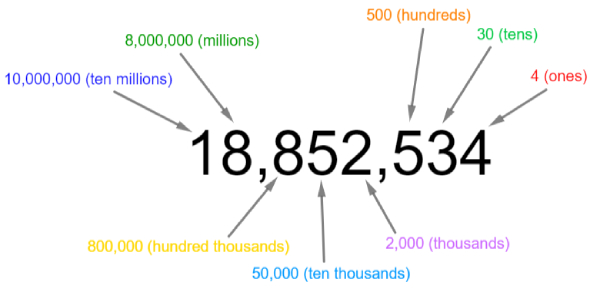# Place Value Exam: Math Quiz

24 Questions | Total Attempts: 349SettingsDo you know anything about place value in Mathematics? Place value supports us in making decisions that are utilized in our everyday life—costs, weight, distances, time, etc. Our number system is based on a base-ten system. Place value refers to the value of each digit in a number. It is an essential concept that we all must learn. If you want to know more, try this quiz.

• 1.
Write the standard form of each. 4000 + 20 + 8 =
• 2.
Write the standard form of each. 16 billion, 6 million, 842 thousand, 301 =
• 3.
Write the standard form of each. 78 million, 4 thousand, 76 =
• 4.
Write the standard form of each. Three and seventeen hundredths =
• 5.
Write the standard form of each.One hundred eleven and one hundred four thousandths =
• 6.
Write the word form for each7812
• 7.
Write the word form for each210.5
• 8.
Write the value of the underlined digit89,106
• 9.
Write the value of the underlined digit398,645
• 10.
Write the value of the underlined digit63,999
• 11.
Write the value of the underlined digit1,459.3
• 12.
Compare27,396 __________ 27,269
• A.

• B.

>

• C.

=

• 13.
Compare.14.76 ________14.761
• A.

• B.

>

• C.

=

• 14.
Compare.8,000 ______ 8 thousand
• A.

• B.

>

• C.

=

• 15.
Round each number to the underlined place value.53, 900
• 16.
Round each number to the underlined place value.584, 978
• 17.
Round each number to the underlined place value.133.123
• 18.
Round each number to the underlined place value.2,634,298
• 19.
Order these from least to greatest.13,242; 11,221; 13,144
• 20.
Order these from least to greatest.109,817; 109,871; 109,877
• 21.
Order these from least to greatest.14.123; 14.124; 15.653
• 22.
The table below shows the revolution of 4 planets. Revolution is the length of time it takes to orbit once around the sun. Use the tables to answer questions 23 and 24.Planet: Revolution in DaysJupiter 4,332 Pluto 90,465Venus 225Uranus 30,685Which planet takes the least number of days to revolve around the sun?
• 23.
The table below shows the revolution of 4 planets. Revolution is the length of time it takes to orbit once around the sun. Use the tables to answer questions 23 and 24.Planet: Revolution in DaysJupiter 4,332 Pluto 90,465Venus 225Uranus 30,685Which planet takes the greatest number of days to revolve around the sun?
• 24.
Write this number in as many forms as you can.2,089
Related TopicsBack to top